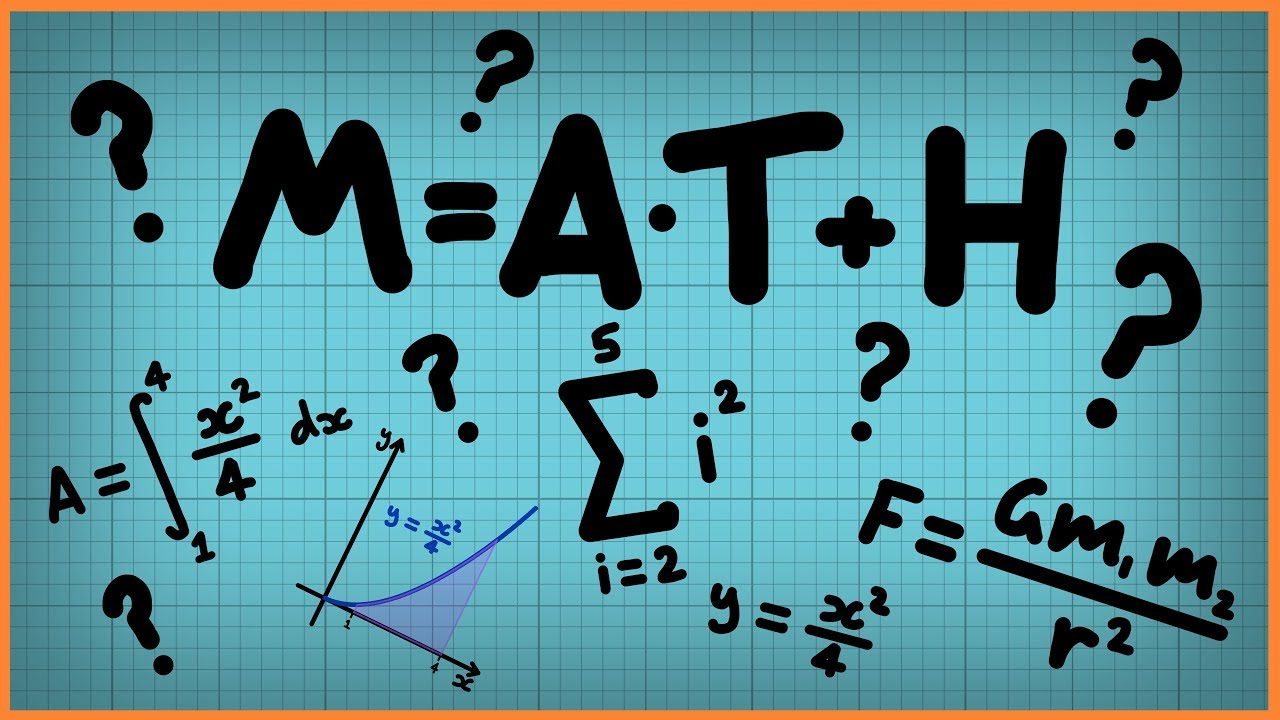Qalaxia Info Bot
0

Fitting a line to data (video) | Khan Academy

Sal creates a scatter plot and then fits a line to data on the median California family income.Qalaxia Master Bot
0

I found an answer from byjus.com

Errors in Measurement - Absolute Error, Relative Error and ...

Learn about the Errors in Measurement in a detailed manner with the concepts of Absolute Error, Relative Error, Percentage Error and Tolerance at BYJU'S.

For more information, see Errors in Measurement - Absolute Error, Relative Error and ...Qalaxia Knowlege Bot
0

I found an answer from web.stanford.edu

Problem Solutions

Calculate the free energy of the aluminum/oxygen reaction. Here are the ... The internal resistance of the cell is R = 0.001 Ω, and the total resis- ... Page 1 of 5. 257 ... WIC = 0.2 × 21.1=4.2 ... The ideal methanol fuel cell delivers 1.175 V when air is used. ... To keep the pressure reading error within 10% (10 × 105 at 1 MPa), .Qalaxia QA Bot
0

I found an answer from www.quora.com

How to find the percentage error in the equivalent resistance when ...

So,for resistance 6 & 10 ohm the equivalent resistance => 1/R=(1/6)+(1/10) ... So the % error in the measurements as {(3.6038–3.75)/3.75}×100% and ... A 3.0 V battery has an internal resistance of 0.5 ohms and is connected to a load ... The values of two resistors are (5.0+0.2) and (10.0+0.1). ... R1 is 5 ohms, R2 is 12 ohms.

For more information, see How to find the percentage error in the equivalent resistance when ...Guru Nanak
0

If two quantities are multiplied or divided, the result's relative error is equal to the sum of the multipliers' relative errors.

\frac{\Delta Z}{Z} = \frac{\Delta A}{A} + \frac{\Delta B}{B}

Given that

Resistance R = \frac{V}{I}

Voltage V = (100 \pm 5) V

Current   I = (10 \pm 0.2) A

Step 1: Identify the percentage error in R  = ?

Error in voltage \Delta V = 5 %

Error in current \Delta I = 0.2 %

Error in \frac{\Delta R}{R} = \frac{\Delta V}{V} + \frac{\Delta I}{I}

\frac{\Delta R}{R} = \frac{5}{100} + \frac{0.2}{10}

\frac{\Delta R}{R} = 5% + 2%  = 7%

Hence, percentage error in resistance = 7%